# Reduce in Swift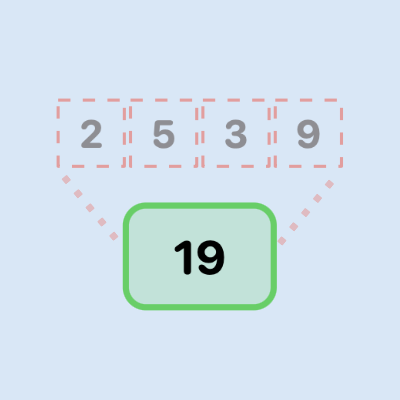Reduce is a simple way to perform an operation over each element in a collection and condense down to a single value. It is commonly used on a list of numeric values to add up all the values.

## Sum all numbers in an Array

The verbose syntax of Reduce takes two parameters, the first being the initial value and the second is a closure taking two parameters. In the Closure; the first parameter is the previous value (or a starter value) and the second parameter is the current value in the collection. The operation is applied between these two values, which becomes the value to feed into the next iteration. The operation is repeated until the end of the collection is reached.

1let nums = [1, 2, 3, 4, 5]
2
3let total1 = nums.reduce(0, { x, y in
4    x + y
5})


The syntax above is rarely used, as it is more common to use the trailing closure syntax.

1let total2 = nums.reduce(0) { x, y in x + y }


This can be shortened further, using the shorthand argument names provided automatically by Swift to inline closures. The trailing closure is updated to simply add the first parameter to the second parameter.

1let total3 = nums.reduce(0) { $0 +$1 }


Finally, this can be shortened even further, by eliminating the closure and specifying the operation as the second parameter to the reduce function.

1let total4 = nums.reduce(0, +)


If the operation is multiplication to produce the product of a list of numbers, then it is important to use 1 as the initial value, otherwise the answer will be zero.

1print("Product 0 = \(nums.reduce(0, *))")
2// Product 0 = 0
3
4
5print("Product 1 = \(nums.reduce(1, *))")
6// Product 1 = 120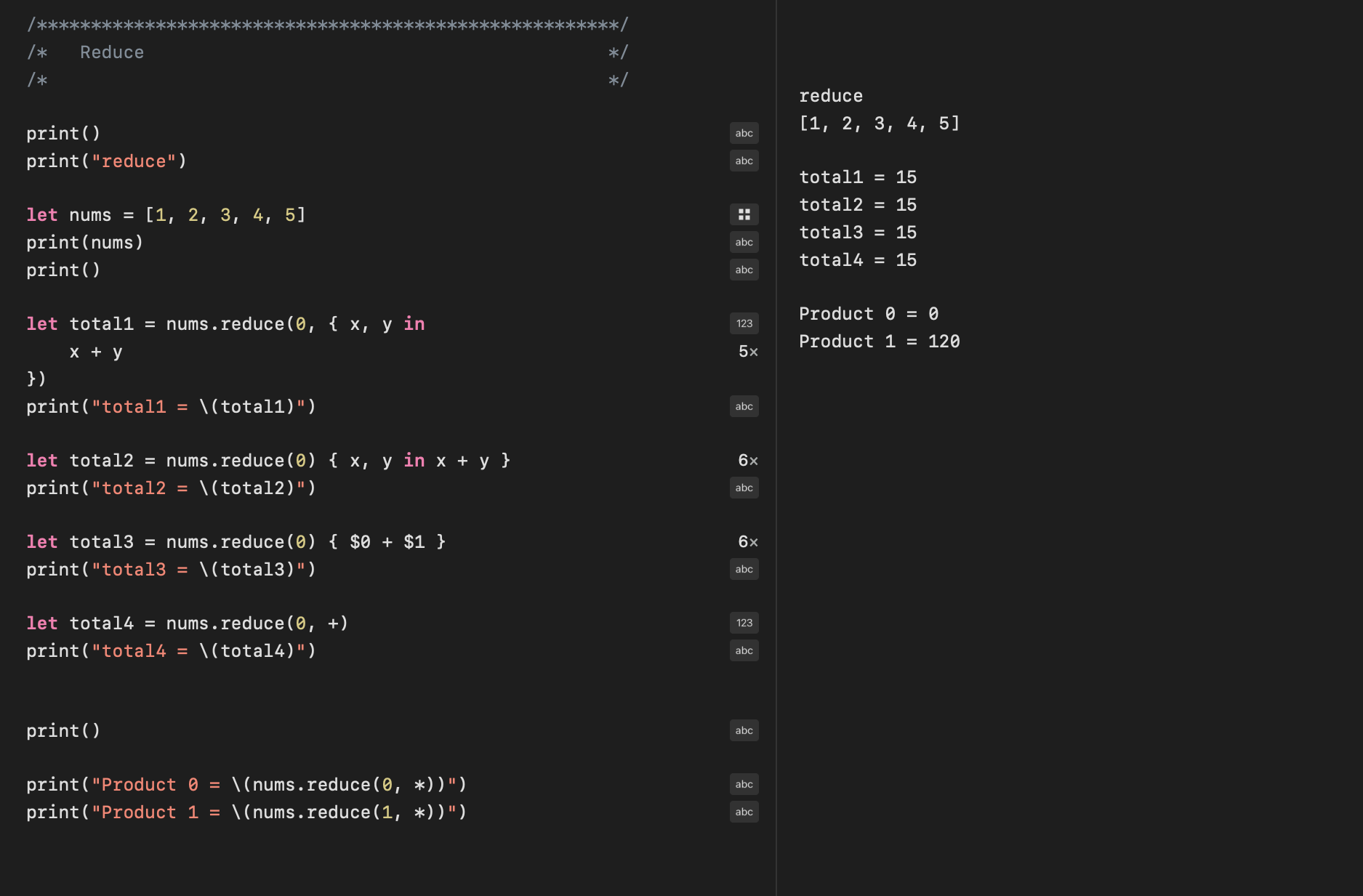Syntax to call reduce on array to add up all the elements

## Concatenate strings together

Reduce can also be used on Strings, where the + operation will concatenation strings. But other mathematical operations are not valid on strings.

 1let stringsArray = ["Red",
2                    "uce",
3                    " ",
4                    "in",
5                    " ",
6                    "Swi",
7                    "ft",
8                    "UI"]
9
10let string1 = stringsArray.reduce("", +)
11print("string1 = \(string1)")
12
13// string1 = Reduce in SwiftUI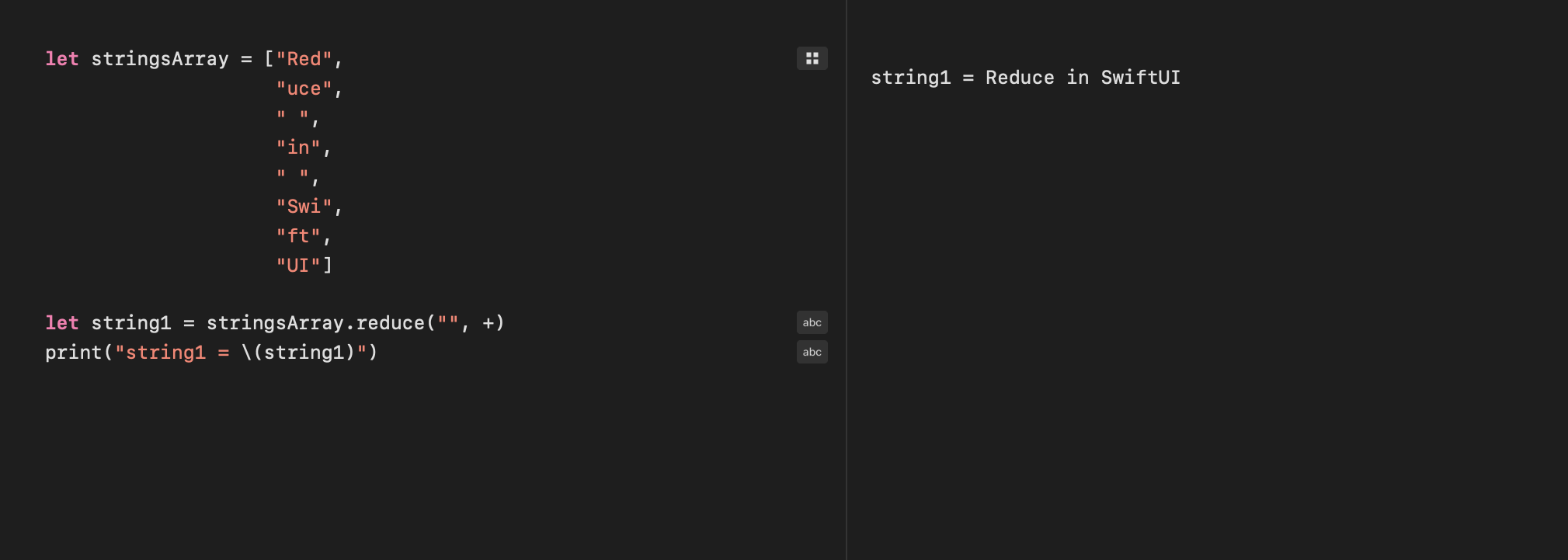Concatenate a list of strings with Reduce

## Cumulative sums with Reduce into

reduce into is used on a sequence to produce a new sequence based on operations performed on the original sequence. This sounds like map except that the operation on each element uses the output from the previous element in the sequence. This creates a sequence of cumulative sums from a sequence of numbers.

1let nums = [1, 2, 3, 4, 5]
2
3let totals1 = nums.reduce(into: [Int](), { x, y in
4    x.append((x.last ?? 0) + y)
5})


The second parameter is more commonly moved to a trailing closure.

1let totals2 = nums.reduce(into: [Int]()) { x, y in
2    x.append((x.last ?? 0) + y)
3}


This can also be shortened further using the shorthand argument names in closures. The trailing closure is updated to add the current element to the previous result and append it to the new sequence.

1let totals3 = nums.reduce(into: [Int]()) {
2    $0.append(($0.last ?? 0) + $1) 3}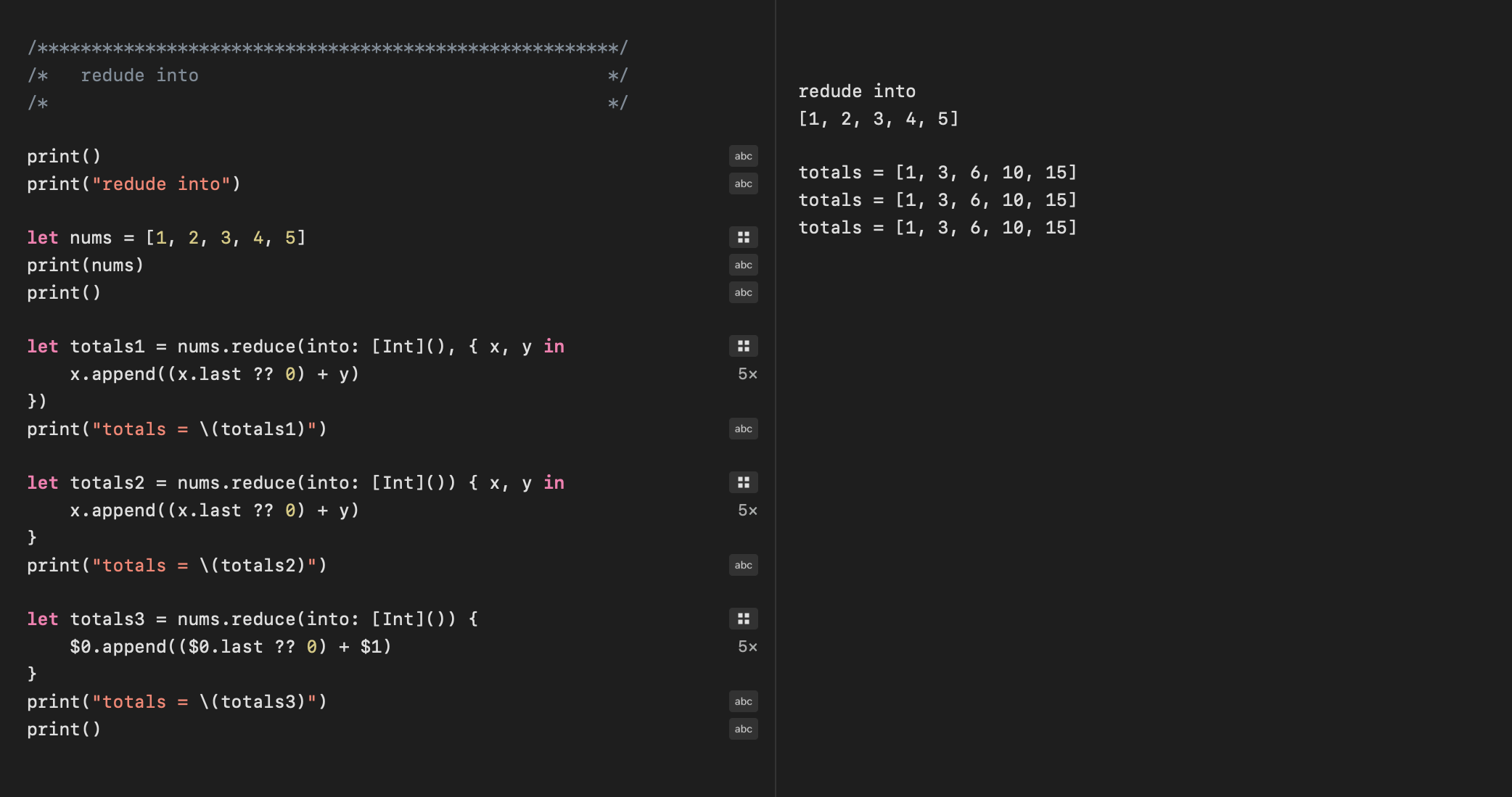Cumulative sum on a sequence of numbers with Reduce(into) ## Removing repeating elements with Reduce into reduce into can also be used to remove repeating elements from a sequence. This code compares the current element with the previous element and only adds it if the element is different. On a sorted sequence, this has the effect of removing duplicates, which could be done more easily using Set. Note that removing repeated elements will not remove all duplicates when the sequence is not sorted and this may be the desired behavior in some circumstances. 1let fullList = [1, 1, 2, 2, 2, 3, 4, 4, 5, 5, 6] 2 3let unique = fullList.reduce(into: [Int]()) { 4 if$0.last != $1 {$0.append($1) } 5}  1let unique2 = Set(fullList).sorted() 2print("unique list = \(unique2)")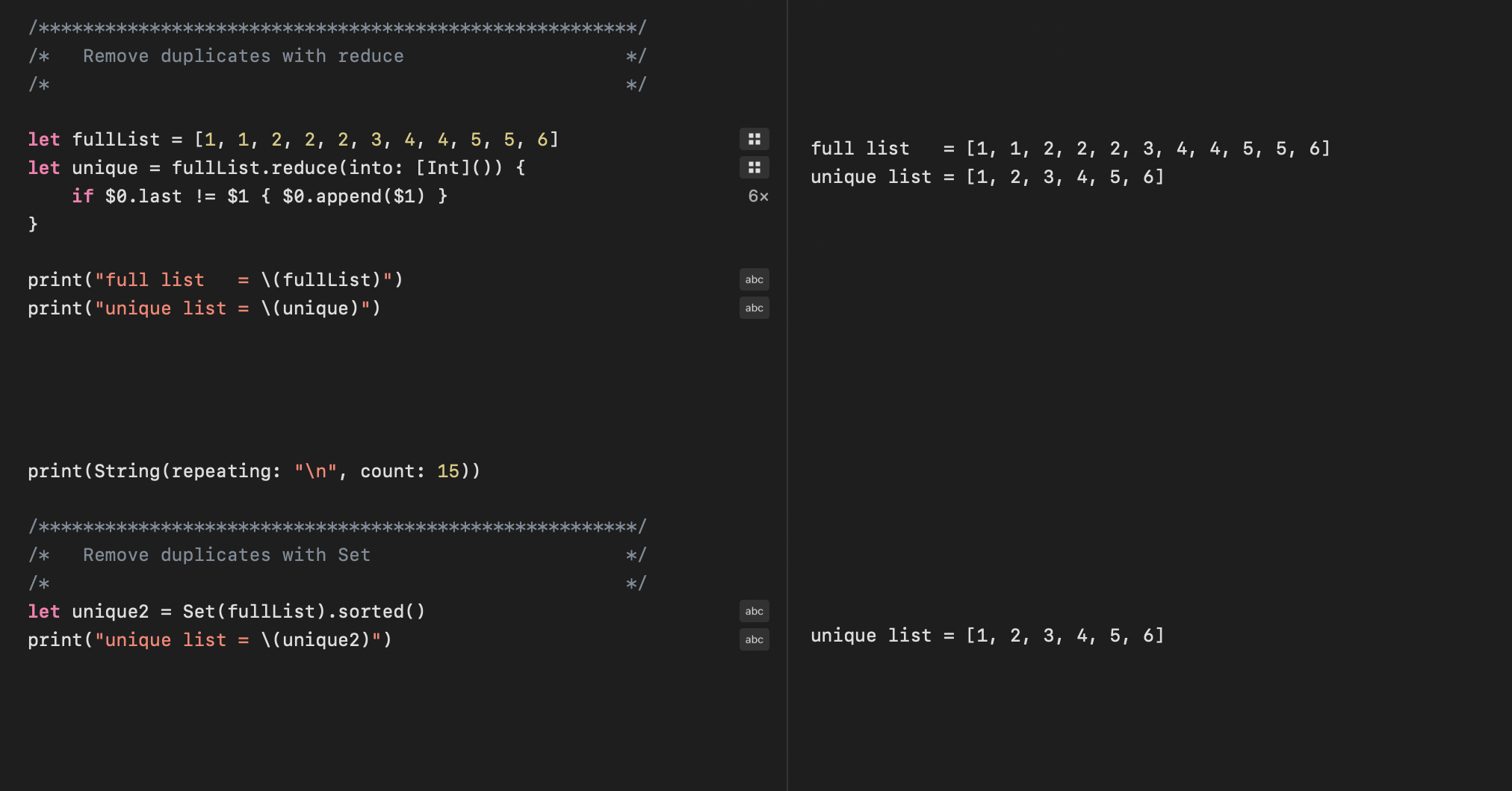Use Reduce(into) to remove repeating elments from a sequence ## Reduce vs Map for incremental differences Reduce can be used to get the incremental difference between elements in a sequence of data that is increasing as the sequence progresses. However, this requires two reduce into operations; the first to create tuples of the current element and the previous element; and the second reduce to calculate the difference between these elements. Reduce is not the best approach for this task and zip and map provide a better solution. 1let data = [5, 6, 8, 15, 17, 21, 23, 23, 24, 32, 34, 37, 39, 40] 2 3let differences = data.reduce(into: [(Int, Int)]()) { 4$0.append((($0.last?.1 ?? 0),$1))
5}
6.reduce(into: [Int]()) {
7    $0.append($1.1 - $1.0) 8}  1let data2 =  + data[..<(data.count - 1)] 2let differences2 = zip(data, data2).map() {$0.0 - \$0.1 }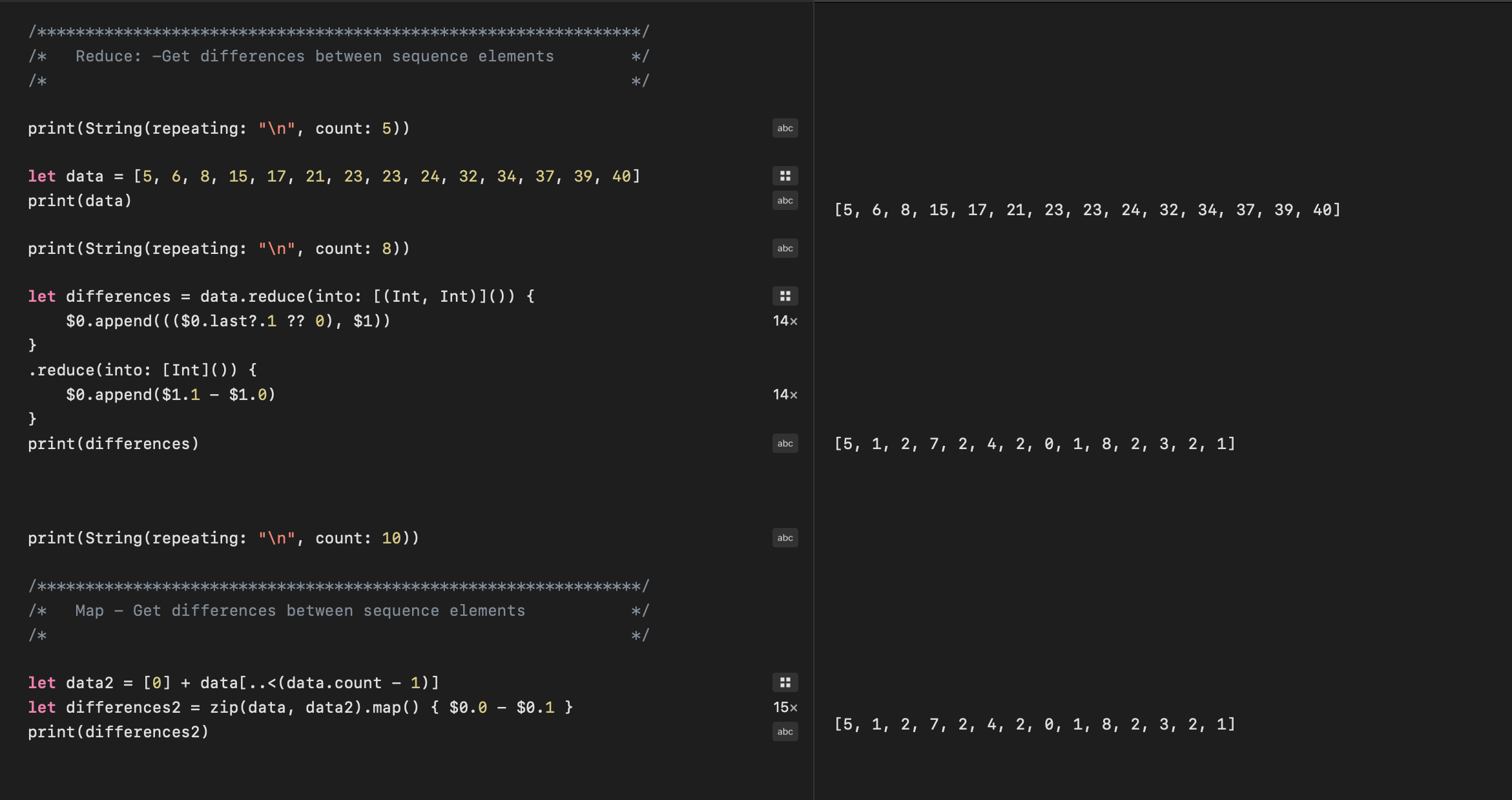Reduce can be used to calculate element differences, but map seems more appropriate

## Conclusion

Reduce is great to quickly add all the numeric elements in a collection. It can be used to perform more complicated tasks such as eliminating duplicate elements from an array, but there are easier ways to do these tasks. Reduce is more frequently used in combination with other transforms such as map and filter and is an important step in transforming data.